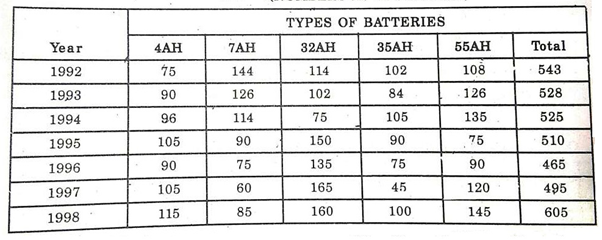• Save

# Data Interpretation Questions with Solutions

7 months ago 2.9K ViewsData interpretation is an essential part of quantitative aptitude. Basically, data interpretation is a combination topic of the bar graph, line graph, tabulation and pie chart questions. But here I am sharing you, data interpretation questions with solutions related to bar graph and tabulation.

With the help of these questions, you can improve your performance and skills to solve data interpretation topic easily. So, continue your practice with these important questions with solutions.

Here you can practice with related to above all data interpretation topic questions and answers.

## Data Interpretation Problems with Solutions

Direction(1-5). The following table gives the sales of batteries manufactured by company over the years. Study the table and answer the question that follows:

NUMBER OF DIFFERENT TYPES OF BATTERIES SOLD BY A COMPANY OVER THE YEARS (NUMBERS IN THOUSAND)Q.1. The total sales of all the seven years is the maximum for which battery?

(A) 4AH

(B) 7AH

(C) 32AH

(D) 35AH

(E) 55AH

Ans .   C

The total sales (in thousand) of all the seven years for various batteries are :

For 4AH = 75 + 90 + 96 + 105 + 90 + 105 + 115 = 676

For 7AH =  144 + 126 + 114 + 90 + 75 + 60 + 85 = 694

For 32AH = 114 + 102 + 75 + 150 + 135 + 165 + 160 = 901

For 35AH =  102 + 84 + 105 + 90 + 75 + 45 + 100 = 601

For 55AH =  108 + 126 + 135 + 75 + 90 + 120 + 145 = 799

Clearly, sales are maximum in case of 32AH batteries

Q.2. What is the difference in the number of 35AH batteries sold in 1993 and 1997 ?

(A) 24000

(B) 28000

(C) 35000

(D) 39000

(E) 42000

Ans .   D

Required difference = [(84 – 45)× 1000]=3900.

Q.3. The percentage of 4AH batteries sold to the total number of batteries sold was maximum in the year :

(A) 1994

(B) 1995

(C) 1996

(D) 1998

Ans .   D

The percentage of sales of 4AH batteries to the total sales in different years are :

For 1992 = $$\left( {75\over 543}×100 \right)\% = 13.81\%$$

For 1993 = $$\left( {92\over 528}×100 \right)\% = 17.05\%$$

For 1994 =   $$\left( {96\over 525}×100 \right)\% = 18.29\%$$

For 1995 = $$\left( {105\over 510}×100 \right)\% = 20.59\%$$

For 1996 = $$\left( {96\over 465}×100 \right)\% = 19.35\%$$

For 1997 =   $$\left( {105\over 495}×100 \right)\% = 21.21\%$$

For 1998 =   $$\left( {115\over 605}×100 \right)\% = 19.01\%$$

Clearly, the percentage is maximum in 1997.

Q.4. In the case of which battery there was a continuous decrease in sales from 1992 to 1997 ?

(A) 4AH

(B) 7AH

(C) 32AH

(D) 35AH

(E) 55AH

Ans .   B

From the table it is clear that the sales of 7AH batteries have been decreasing continuously from 1992 to 1997.

Q.5. What was the approximate percentage increase in the sales of 55AH batteries in 1998 compared to that in 1992 ?

(A) 28%

(B) 31%

(C) 33%

(D) 34%

(E) 37%

Ans .   D

Required Percentage = $$\left( {145-108\over 108}×100 \right)\% = 34.26\%$$

Direction(6-10): The bar graph given shows the foreign exchange reserves of a country (in million US $) form 1991-92 to 1998-99. Answer the question based on this graph.Q.6. The foreign exchange reserves in 1997-98 was how many times that in 1994-95? (A) 0.7 (B) 1.2 (C) 1.4 (D) 1.5 (E) 1.8 Ans . D Required ration $$= {5040\over 3360}=1.5$$ Q.7. What was the percentage increase of foreign exchange reserves over the previous year, is the highest? (A) 100 (B) 150 (C) 200 (D) 620 (E) 2520 Ans . A Foreign exchange reserves in 1997-98 = 5040 million US$

Foreign exchange reserves in 1993-94 = 2520 million US$⸫ Increase = (5040 – 2520) = 2520 million US$

⸫ Percentage increase = $$= \left({2520\over 2520}× 100\right)\% = 100\%$$

Q.8. For which year, the percentage increase of foreign exchange reserves over the previous year, is the highest?

(A) 1992-93

(B) 1993-94

(C) 1994-95

(D) 1996-97

(E) 1997-98

Ans .   A

There is an increase in foreign exchange reserves during the years 1992-93, 1996-97 and 1997-98 as compared to previous year (as shown by bar-graoh).

The percentage increase in reserves during these years compared to previous year are :

(i) For 1992-93   $$= \left({(3720-2640)\over 2640}× 100\right)\% = 40.91\%$$

(ii) For 1994-95 $$= \left({(3360-2520)\over 2520}× 100\right)\% = 33.33\%$$

(iii) For 1996-97  $$= \left({(4320-3120)\over 3120}× 100\right)\% = 38.46\%$$

(iv) For 1997-98  $$= \left({(5040-4320)\over 4320}× 100\right)\% = 16.67\%$$

Clearly, the percentage increase over previous year is highest for 1992-93.

Q.9. The foreign exchange reserves in 1996-97 were approximately what percent of the average foreign exchange reserves over the period under review?

(A) 95%

(B) 110%

(C) 115%

(D) 125%

(E) 140%

Ans .   D

Average foreign exchange reserves over the given period

$$=\left({1\over 8}× (2640+3720+2520+3360+3120+4320+5040+3120) \right)$$

= 3480 million US $. Foreign exchange reserves in 1996 – 97 = 4320 million US$.

⸫ Required Percentage =   $$= \left({4320\over 3480}× 100\right)\% = 124.14\%$$

Q.10. The ratio of the number of years, in which the foreign exchange reserves are above the average reserves, to those in which the reserves are below the average reserves, is:

(A) 2 : 6

(B) 3 : 4

(C) 3 : 5

(D) 4 : 4

(E) 5 : 3

Ans .   C

Average foreign exchange reserves over the given period= 3480 million US $. The country had reserves above 3480 million US$ during the years 1992-93, 1996-97 and 1997-98 i.e., for 3 years and below 3480 million US \$ during the years 1991-92, 1993-94, 1994-95, 1995-96 and 1998-99 i.e., for 5 years.

Hence, required ration = 3 : 5